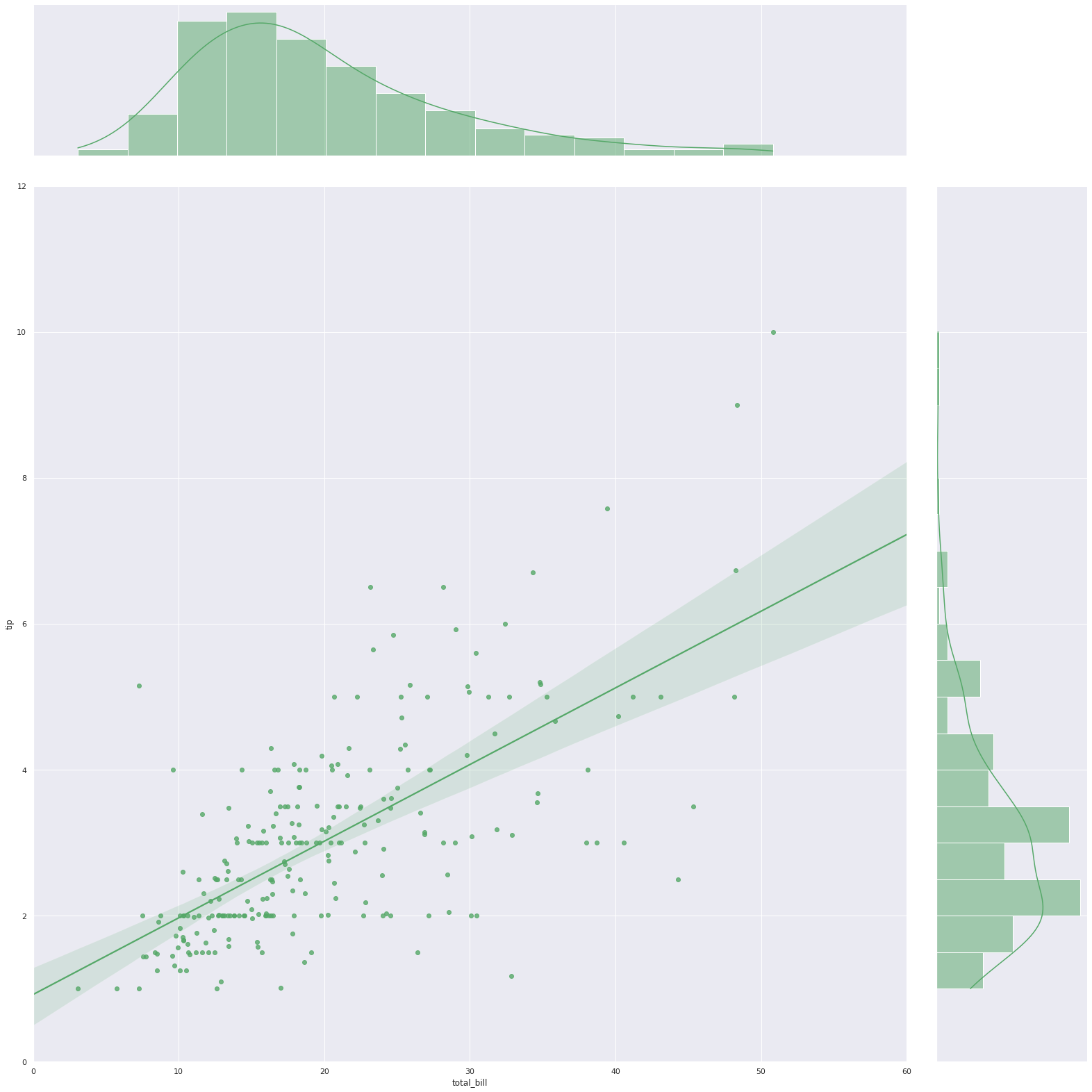# Linear Regression for Tip vs Total Bill

Dec 03, 2021

## Linear Regression

Linear regression is a linear approach for modelling the relationship between a scalar response and one or more explanatory variables (also known as dependent and independent variables). The case of one explanatory variable is called simple linear regression; for more than one, the process is called multiple linear regression. This term is distinct from multivariate linear regression, where multiple correlated dependent variables are predicted, rather than a single scalar variable.

In linear regression, the relationships are modeled using linear predictor functions whose unknown model parameters are estimated from the data. Such models are called linear models. Most commonly, the conditional mean of the response given the values of the explanatory variables (or predictors) is assumed to be an affine function of those values; less commonly, the conditional median or some other quantile is used. Like all forms of regression analysis, linear regression focuses on the conditional probability distribution of the response given the values of the predictors, rather than on the joint probability distribution of all of these variables, which is the domain of multivariate analysis.

Linear regression was the first type of regression analysis to be studied rigorously, and to be used extensively in practical applications. This is because models which depend linearly on their unknown parameters are easier to fit than models which are non-linearly related to their parameters and because the statistical properties of the resulting estimators are easier to determine.

## Linear Regression Practical uses

Linear regression has many practical uses. Most applications fall into one of the following two broad categories:

1. If the goal is prediction, forecasting, or error reduction, linear regression can be used to fit a predictive model to an observed data set of values of the response and explanatory variables. After developing such a model, if additional values of the explanatory variables are collected without an accompanying response value, the fitted model can be used to make a prediction of the response.

2. If the goal is to explain variation in the response variable that can be attributed to variation in the explanatory variables, linear regression analysis can be applied to quantify the strength of the relationship between the response and the explanatory variables, and in particular to determine whether some explanatory variables may have no linear relationship with the response at all, or to identify which subsets of explanatory variables may contain redundant information about the response.

## Formulation

Given a data set, $${y_{i},,x_{i1},\ldots ,x_{ip}}{i=1}^{n}}{y{i},,x_{i1},\ldots ,x_{ip}}_{i=1}^{n}$$ of n statistical units, a linear regression model assumes that the relationship between the dependent variable y and the p-vector of regressors x is linear. This relationship is modeled through a disturbance term or error variable ε — an unobserved random variable that adds “noise” to the linear relationship between the dependent variable and regressors.

## BILL VS TIP

Tip is a sum of money customarily given by a customer to certain service sector workers for the service they have performed, in addition to the basic price of the service.

## TOTAL BILL VS TOTAL TIP - Classic Example of Linear Regression

The figure shows that there is some positive linear relationship between the total bill paid by the restaurant customers and the tip amount given to waiters.

Thus the model takes the form, $$y_{i}=\beta _{0}+\beta {1}x{i1}+\cdots +\beta {p}x{ip}+\varepsilon _{i}=\mathbf {x} _{i}^{\mathsf {T}}{\boldsymbol {\beta }}+\varepsilon {i},\qquad i=1,\ldots ,n,}y{i}=\beta _{0}+\beta {1}x{i1}+\cdots +\beta {p}x{ip}+\varepsilon _{i}=\mathbf {x} _{i}^{\mathsf {T}}{\boldsymbol {\beta }}+\varepsilon _{i},\qquad i=1,\ldots ,n,}$$ where T denotes the transpose, so that xiTβ is the inner product between vectors xi and β.

$$\mathbf {y} =X{\boldsymbol {\beta }}+{\boldsymbol {\varepsilon }},,}$$